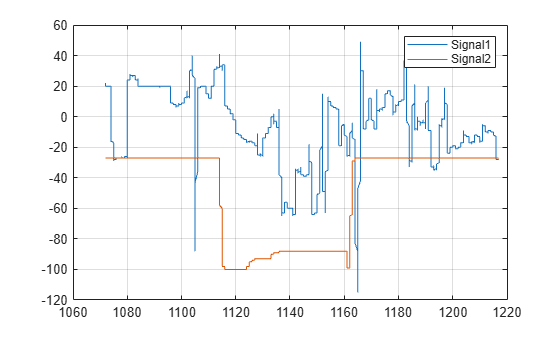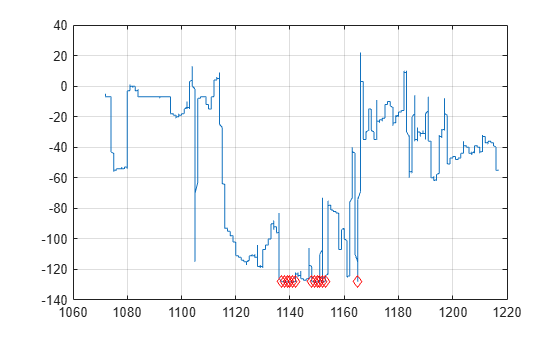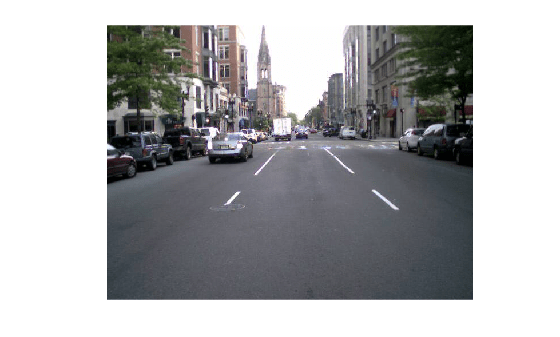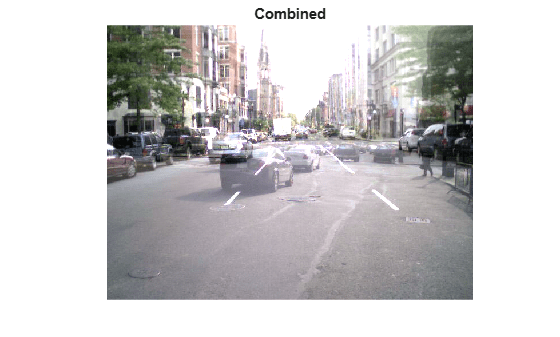# Integer Arithmetic

This example shows how to perform arithmetic on integer data representing signals and images.

Load measurement datasets comprising signals from four instruments using 8 and 16-bit A-to-D's resulting in data saved as `int8`, `int16` and `uint16`. Time is stored as `uint16`.

```load integersignal % Look at variables whos Signal1 Signal2 Signal3 Signal4 Time1```
``` Name Size Bytes Class Attributes Signal1 7550x1 7550 int8 Signal2 7550x1 7550 int8 Signal3 7550x1 15100 int16 Signal4 7550x1 15100 uint16 Time1 7550x1 15100 uint16 ```

### Plot Data

First we will plot two of the signals to see the signal ranges.

```plot(Time1, Signal1, Time1, Signal2); grid; legend('Signal1','Signal2');```It is likely that these values would need to be scaled to calculate the actual physical value that the signal represents e.g. Volts.

### Process Data

We can perform standard arithmetic on integers such as `+`, `-`, `*`, and `/`. Let's say we wished to find the sum of Signal1 and Signal2.

`SumSig = Signal1 + Signal2; % Here we sum the integer signals.`

Now let's plot the sum signal and see where it saturates.

```cla; plot(Time1, SumSig); hold on Saturated = (SumSig == intmin('int8')) | (SumSig == intmax('int8')); % Find where it has saturated plot(Time1(Saturated),SumSig(Saturated),'rd') grid hold off```The markers show where the signal has saturated.

Next we will look at arithmetic on some image data.

```street1 = imread('street1.jpg'); % Load image data street2 = imread('street2.jpg'); whos street1 street2```
``` Name Size Bytes Class Attributes street1 480x640x3 921600 uint8 street2 480x640x3 921600 uint8 ```

Here we see the images are 24-bit color, stored as three planes of `uint8` data.

### Display Images

Display first image.

```cla; image(street1); % Display image axis equal axis off```Display second image

```image(street2); % Display image axis equal axis off```### Scale an Image

We can scale the image by a double precision constant but keep the image stored as integers. For example,

```duller = 0.5 * street2; % Scale image with a double constant but create an integer whos duller```
``` Name Size Bytes Class Attributes duller 480x640x3 921600 uint8 ```
```subplot(1,2,1); image(street2); axis off equal tight title('Original'); % Display image subplot(1,2,2); image(duller); axis off equal tight title('Duller'); % Display image```We can add the two street images together and plot the ghostly result.

```combined = street1 + duller; % Add |uint8| images subplot(1,1,1) cla; image(combined); % Display image title('Combined'); axis equal axis off```